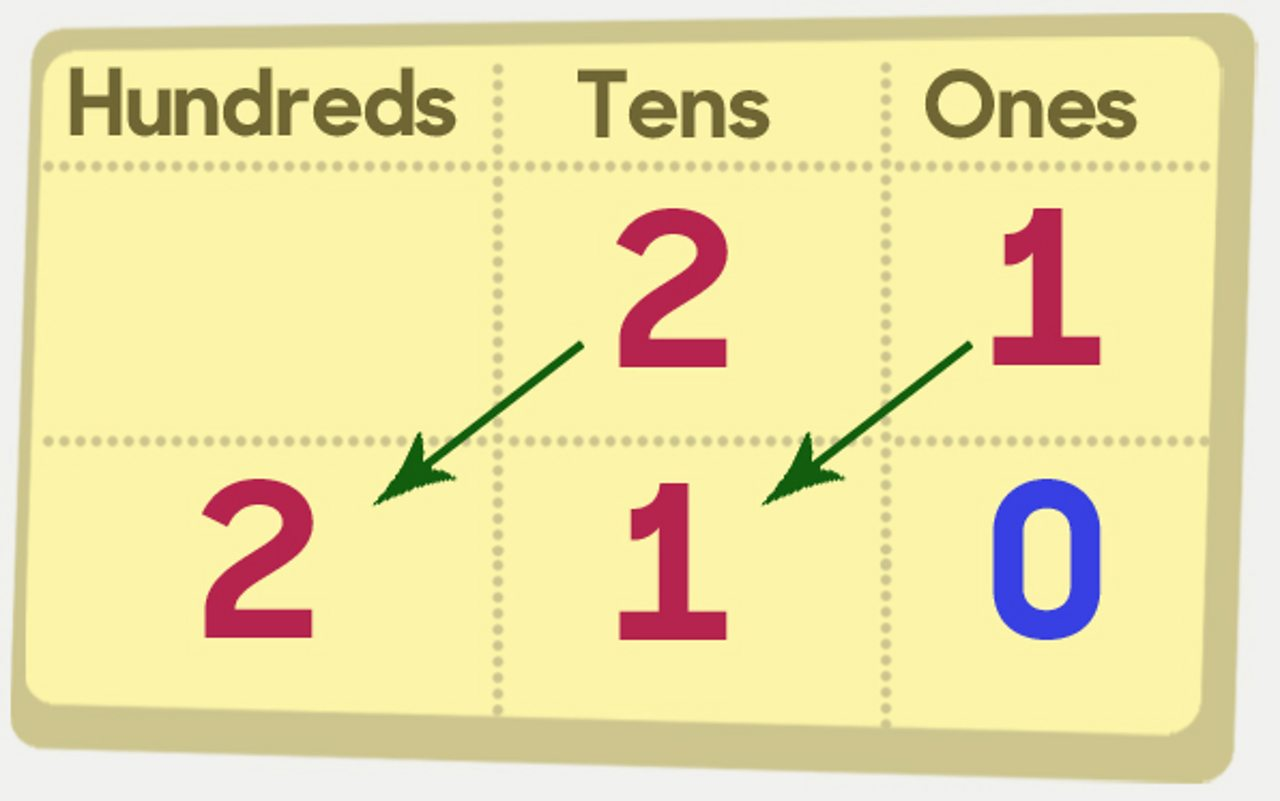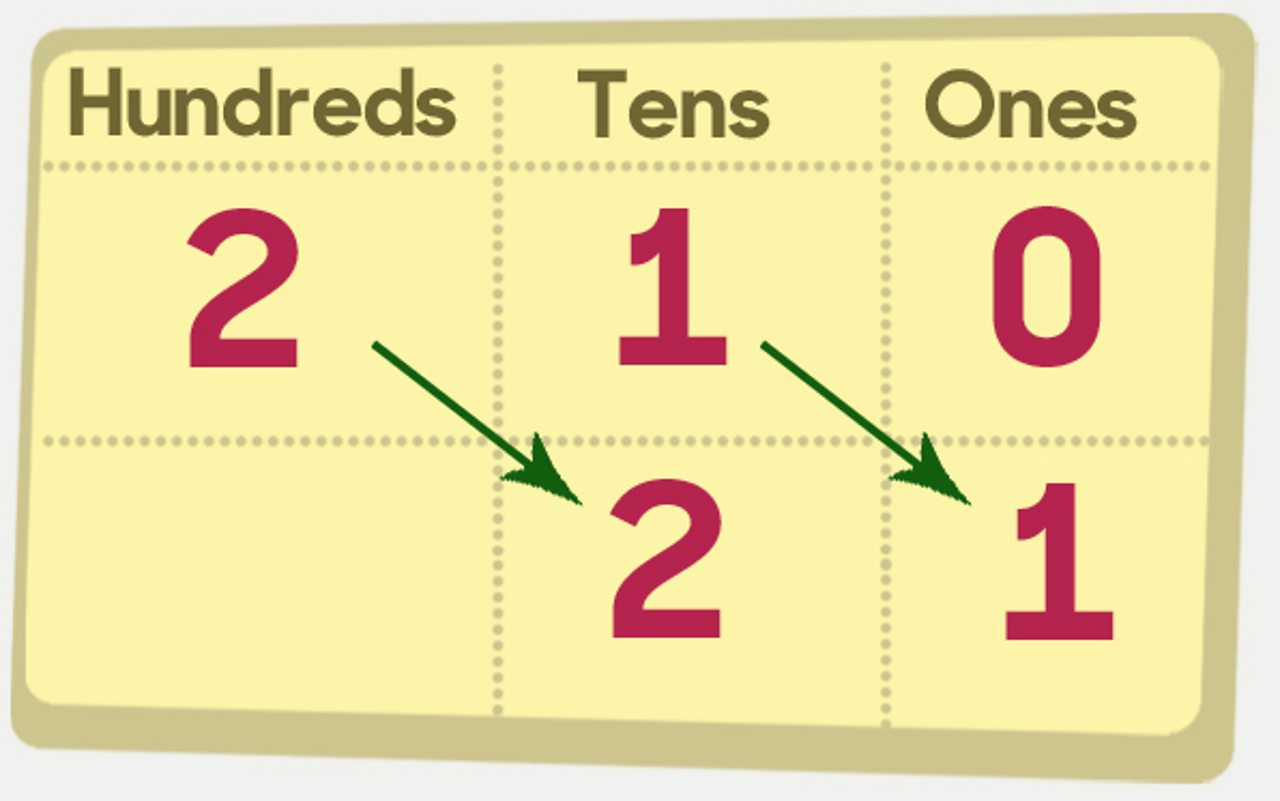# How to multiply and divide by 0, 1, 10 and 100

Multiplying by 1, 10, or 100

• When you multiply by 1 the answer stays the same. 21 × 1 = 21
• When you multiply by 10, move all the digits one place to the left, putting a zero in the empty space. 21 × 10 = 210
• When you multiply by 100, move all the digits two places to the left, putting a zero in the empty spaces. 21 × 100 = 210021 × 10 = 210

Any number multiplied by 0 is 0

• 5 × 0 = 0
• 100 × 0 = 0
• 9456 × 0 = 0
• What is 1,000,000 × 0?

Dividing by 1, 10 or 100

• When you divide by 1 the answer stays the same. 21 ÷ 1 = 21
• When you divide by 10, move all the digits one place to the right. 210 ÷ 10 = 21
• When you divide by 100, move all the digits two places to the right. 2100 ÷ 100 = 21210 ÷ 10 = 21